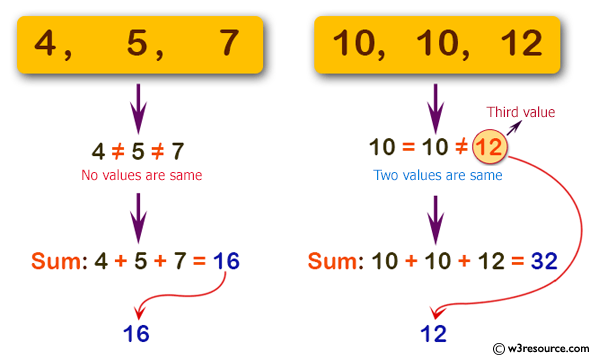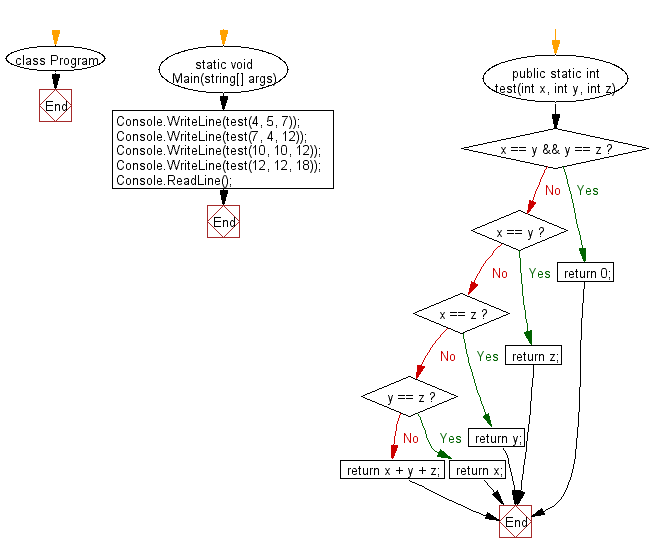﻿ C# - Sum 3 integers, if 2 values are same, return the third# C# Sharp Basic Algorithm Exercises: Compute the sum of three given integers. If the two values are same return the third value

## C# Sharp Basic Algorithm: Exercise-55 with Solution

Write a C# Sharp program to compute the sum of three given integers. If the two values are same return the third value.

Pictorial Presentation:Sample Solution:-

C# Sharp Code:

``````using System;
using System.Linq;
namespace exercises
{
class Program
{
static void Main(string[] args)
{
Console.WriteLine(test(4, 5, 7));
Console.WriteLine(test(7, 4, 12));
Console.WriteLine(test(10, 10, 12));
Console.WriteLine(test(12, 12, 18));
}
public static int test(int x, int y, int z)
{
if (x == y && y == z) return 0;
if (x == y) return z;
if (x == z) return y;
if (y == z) return x;
return x + y + z;
}
}
}
```
```

Sample Output:

```16
23
12
18```

Flowchart:C# Sharp Code Editor:

Improve this sample solution and post your code through Disqus

What is the difficulty level of this exercise?

Test your Programming skills with w3resource's quiz.

﻿